Journal topic
Atmos. Chem. Phys., 20, 6147–6158, 2020
https://doi.org/10.5194/acp-20-6147-2020Atmos. Chem. Phys., 20, 6147–6158, 2020
https://doi.org/10.5194/acp-20-6147-2020Research article 26 May 2020

Research article | 26 May 2020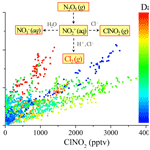# Significant production of ClNO2 and possible source of Cl2 from N2O5 uptake at a suburban site in eastern China

Significant production of ClNO2 and possible source of Cl2 from N2O5 uptake at a suburban site in eastern China
Men Xia1, Xiang Peng1, Weihao Wang1, Chuan Yu1, Peng Sun2, Yuanyuan Li2, Yuliang Liu2, Zhengning Xu2, Zhe Wang1,a, Zheng Xu2, Wei Nie2, Aijun Ding2, and Tao Wang1 Men Xia et al.
• 1Department of Civil and Environmental Engineering, The Hong Kong Polytechnic University, Hong Kong SAR, China
• 2Joint International Research Laboratory of Atmospheric and Earth System Sciences, School of Atmospheric Sciences, Nanjing University, Nanjing, 210023, China
• anow at: Division of Environment and Sustainability, Hong Kong University of Science and Technology, Hong Kong SAR, China

Correspondence: Tao Wang (cetwang@polyu.edu.hk)

Abstract

ClNO2 and Cl2 can affect atmospheric oxidation and thereby the formation of ozone and secondary aerosols, yet their sources and production mechanisms are not well understood or quantified. In this study we present field observations of ClNO2 and Cl2 at a suburban site in eastern China during April 2018. Persistent high levels of ClNO2 (maximum: ∼3.7 ppbv; 1 min average) were frequently observed at night, due to the high ClNO2 yield (φ (ClNO2), 0.56±0.20) inferred from the measurements. The φ (ClNO2) value showed a positive correlation with the [Cl] / [H2O] ratio, and its parameterization was improved at low to median yields (0–0.75) by the incorporation of [Cl] / [H2O] and the suppression effect of aerosol organics. ClNO2 and Cl2 showed a significant correlation on most nights. We show that the Cl2 at our site was more likely a co-product with ClNO2 from N2O5 uptake on acidic aerosols that contain chloride than being produced by ClNO2 uptake as previously suggested. We propose a mechanism in which ${\mathrm{NO}}_{\mathrm{2}}^{+}$ can react with Cl to produce Cl2 and ClNO2 simultaneously. Under a new framework which regards Cl2, ClNO2, and nitrate as products of N2O5 uptake, the Cl2 yield (φ (Cl2)) was derived using ambient data. φ (Cl2) exhibited significant correlations with [Cl] and [H+], based on which a parameterization of φ (Cl2) was developed. The derived parameterizations of φ (ClNO2) and φ (Cl2) can be used in models to evaluate the nighttime production of ClNO2 and Cl2 and their impact on the next day's photochemistry.

1 Introduction

Chlorine radicals (Cl) are potent oxidizers in the atmosphere (Seinfeld and Pandis, 2016). Cl destroy the O3 layer in the stratosphere, exposing the biosphere to excess ultraviolet radiation (Molina and Rowland, 1974). In the polluted troposphere, Cl react with volatile organic compounds (VOCs), especially alkanes; contribute to primary ROx (= OH +HO2+RO2) production; and affect hydroxyl radical (OH) and O3 concentrations (Simpson et al., 2015). Nitryl chloride (ClNO2) is a major chlorine radical precursor in the troposphere and has been investigated around the globe over the past decade (Osthoff et al., 2008; Thornton et al., 2010; Mielke et al., 2011; Wang et al., 2016). ClNO2 is an important nocturnal reservoir of chlorine and NOx and is produced mostly at night. NOx reacts with O3 to form NO3 radicals and N2O5 (Reactions R1 and R2). When aerosol chloride is present, ClNO2 and nitrate are produced from the heterogeneous uptake of N2O5 on aerosols (Reaction R3) (Finlayson-Pitts et al., 1989). After sunrise, ClNO2 is photolyzed to return NO2 and release Cl (Reaction R4).

$\begin{array}{}\text{(R1)}& {\mathrm{NO}}_{\mathrm{2}}\left(g\right)+{\mathrm{O}}_{\mathrm{3}}\left(g\right)\to {\mathrm{NO}}_{\mathrm{3}}\left(g\right)+{\mathrm{O}}_{\mathrm{2}}\left(g\right)\text{(R2)}& {\mathrm{NO}}_{\mathrm{3}}\left(g\right)+{\mathrm{NO}}_{\mathrm{2}}\left(g\right)↔{\mathrm{N}}_{\mathrm{2}}{\mathrm{O}}_{\mathrm{5}}\left(g\right)\text{(R3)}& {\mathrm{N}}_{\mathrm{2}}{\mathrm{O}}_{\mathrm{5}}\left(g\right)+{\mathrm{Cl}}^{-}\left(\mathrm{aq}\right)\to {\mathrm{ClNO}}_{\mathrm{2}}\left(g\right)+{\mathrm{NO}}_{\mathrm{3}}^{-}\left(\mathrm{aq}\right)\text{(R4)}& {\mathrm{ClNO}}_{\mathrm{2}}\left(g\right)+hv\to {\mathrm{Cl}}^{\cdot }\left(g\right)+{\mathrm{NO}}_{\mathrm{2}}\left(g\right)\end{array}$

Two key kinetic parameters for quantification of ClNO2 formation are γ (N2O5) (i.e., N2O5 uptake probability on aerosols) and φ (ClNO2) (i.e., ClNO2 production yield from N2O5 uptake) (Thornton et al., 2003; Behnke et al., 1997). Laboratory studies have shown that φ (ClNO2) is dependent on the [Cl] / [H2O] ratio because aqueous Cl and H2O compete for the NO${}_{\mathrm{2}}^{+}$ intermediate, based upon which a parameterization was developed to predict φ (ClNO2) (hereafter denoted as φ (ClNO2)BT) (Bertram and Thornton, 2009). The parameterization was tested in several field studies, and it was found that the parameterized φ (ClNO2) values were significantly larger than the field-derived values (Tham et al., 2016, 2018; Wang et al., 2017; McDuffie et al., 2018b; Staudt et al., 2019). The exact causes of these discrepancies are not fully understood. The suppression of φ (ClNO2) has been observed in biomass-burning plumes in northern China, but the specific species that reduced φ (ClNO2) were not identified (Tham et al., 2018). Some inorganic nucleophiles, such as sulfate, and organic nucleophiles, such as acetate, were recently proposed to decrease φ (ClNO2) by consuming ${\mathrm{NO}}_{\mathrm{2}}^{+}$ (McDuffie et al., 2018b; Staudt et al., 2019). Such ${\mathrm{NO}}_{\mathrm{2}}^{+}$-consuming nucleophiles may generate products from N2O5 uptake other than ClNO2 and nitrate, and this is deserving of further investigation.

Besides ClNO2, Cl2 is another important chlorine radical precursor that is present in the lower troposphere (Spicer et al., 1998; Custard et al., 2016; Priestley et al., 2018). Elevated levels of Cl2 (up to ∼400 pptv) have been observed during the daytime in polar and continental environments (Liao et al., 2014; Liu et al., 2017), whereas other studies found nocturnal peaks of Cl2 mixing ratios in polar, coastal, and continental sites (Mielke et al., 2011; Riedel et al., 2012, 2013; McNamara et al., 2019). Several potential sources of Cl2 have been proposed, such as direct emissions from power plants (Riedel et al., 2013) and water treatment facilities (Mielke et al., 2011), photochemical formation associated with O3 (Liao et al., 2014), photoinduced production by TiO2 (Li et al., 2020), and heterogeneous conversion from chlorinated compounds (Reactions R5 and R6) (Deiber et al., 2004; Pratte and Rossi, 2006; McNamara et al., 2019).

$\begin{array}{}\text{(R5)}& \mathrm{HOCl}\left(g\right)+{\mathrm{H}}^{+}\left(\mathrm{aq}\right)+{\mathrm{Cl}}^{-}\left(\mathrm{aq}\right)\to {\mathrm{Cl}}_{\mathrm{2}}\left(g\right)+{\mathrm{H}}_{\mathrm{2}}\mathrm{O}\text{(R6)}& \begin{array}{rl}{\mathrm{ClONO}}_{\mathrm{2}}\left(g\right)& +{\mathrm{H}}^{+}\left(\mathrm{aq}\right)+{\mathrm{Cl}}^{-}\left(\mathrm{aq}\right)\to {\mathrm{Cl}}_{\mathrm{2}}\left(g\right)\\ & +{\mathrm{HNO}}_{\mathrm{3}}\left(\mathrm{aq}\right)\end{array}\end{array}$

Cl2 can also be produced from heterogeneous N2O5 uptake on acidic aerosols laden with chloride, and ClNO2 (aq) has been proposed as an intermediate in Cl2 production (Reaction R7) on the basis of laboratory studies (Roberts et al., 2008, 2009). Those studies hypothesized that ClNO2 first reacts with H+ to form protonated ClNO2 (${\mathrm{HClNO}}_{\mathrm{2}}^{+}$), which further reacts with Cl to produce Cl2 and HNO2.

$\begin{array}{}\text{(R7)}& {\mathrm{ClNO}}_{\mathrm{2}}\left(\mathrm{aq}\right)+{\mathrm{H}}^{+}\left(\mathrm{aq}\right)+{\mathrm{Cl}}^{-}\left(\mathrm{aq}\right)\to {\mathrm{Cl}}_{\mathrm{2}}\left(g\right)+{\mathrm{HNO}}_{\mathrm{2}}\left(\mathrm{aq}\right)\end{array}$

Significant correlations of ClNO2 and Cl2 were observed during an airborne campaign in the United States and were interpreted as evidence of Cl2 production from ClNO2 uptake on acidic aerosols (Haskins et al., 2019). However, this study also found that Cl2 formation from ClNO2 uptake was less efficient, because the estimated γ (ClNO2) value ((2.3±1.8) $×{\mathrm{10}}^{-\mathrm{5}}$) was 2 orders of magnitude lower than that suggested by laboratory studies ((6.0±2.0) $×{\mathrm{10}}^{-\mathrm{3}}$) (Roberts et al., 2008; Haskins et al., 2019). It remains unclear whether ClNO2 uptake proceeds more slowly in ambient environments than in laboratory conditions or whether additional pathways are responsible for the formation of Cl2. Therefore, the detailed activation process by which inert chlorine (e.g., particulate chloride) is converted to reactive chlorine remains highly uncertain and requires further research.

In April 2018, we conducted field measurements of ClNO2, Cl2, and other trace gases and aerosols in a suburban area of the Yangtze River Delta (YRD), a highly populated and industrialized region in eastern China. High levels of ClNO2 with enhanced Cl2 were observed at night. In this study, we investigated the activation of chlorine initiated by heterogeneous N2O5 chemistry. We first introduce prominent features of the observation results. The key parameters in ClNO2 formation (i.e., γ (N2O5) and φ (ClNO2)) are then derived using the ambient data. Factors that influence φ (ClNO2) are discussed, with a focus on a revision of the parameterization of φ (ClNO2). We present observational evidence for a possible co-production pathway of Cl2 with ClNO2 from heterogeneous reactions of N2O5 and propose a new parameterization for nocturnal formation of Cl2.

2 Methods

## 2.1 Observation sites

The field campaign was conducted from 11 to 26 April 2018 on the Xianlin Campus of Nanjing University, which is situated in a suburban area approximately 20 km northeast of downtown Nanjing (see Fig. 1). The observation sites are surrounded by teaching and residential buildings, sparse roads, and vegetation cover for about 1 to 2 km, with no significant emission sources. Approximately 15 km northwest of the sampling sites are large-scale chemical and steel facilities, which can be sources of gaseous pollutants (CO, SO2, NOx, and VOCs) and particulate matters that may influence the site (Zhou et al., 2017). In addition, Shanghai is approximately 270 km southeast of the measurement site.

The main data reported in this study (i.e., N2O5, ClNO2, and Cl2) and the NOx and O3 data were obtained at the School of Atmospheric Sciences (SAS) of Nanjing University (sampling site 1). The auxiliary data – including O3, VOCs, aerosol size distribution, and chemical composition – were obtained at the Station for Observing Regional Processes of the Earth System (SORPES, sampling site 2). Figure 1 shows the locations of the two sampling sites. Interested readers are referred to previous studies for more information about the SORPES site (e.g., Ding et al., 2013, 2019; Sun et al., 2018). A comparison of O3 measurements at the SAS and SORPES sites shows excellent agreement during the observation period (Fig. S1 in the Supplement).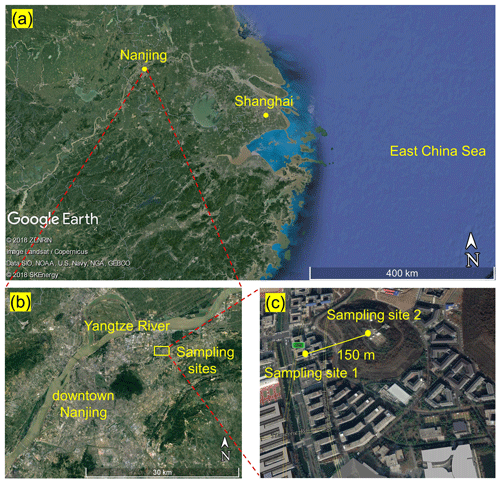Figure 1Sampling locations. (a) Location of Nanjing city in the YRD region. (b) Location of sampling sites in Nanjing. (c) Sampling sites 1 and 2 on the Xianlin Campus of Nanjing University.

## 2.2N2O5, ClNO2, and Cl2 measurements

A chemical ionization mass spectrometer coupled with a quadrupole mass analyzer (Q-CIMS, THS Instruments) was used to detect N2O5, ClNO2, Cl2, and HOCl. The Q-CIMS had been used in previous field campaigns to measure N2O5 and ClNO2 (Wang et al., 2016; Tham et al., 2016). In this study, we also measured Cl2 and HOCl and tuned the pressure of the drift tube reactor accordingly. The principles and ion chemistry of Q-CIMS were described in detail by Kercher et al. (2009). Briefly, iodide (I) was adopted as the primary ion for strong affinity with our target species. Charged iodide clusters – such as ${\mathrm{IN}}_{\mathrm{2}}{\mathrm{O}}_{\mathrm{5}}^{-}$, ${\mathrm{IClNO}}_{\mathrm{2}}^{-}$, ${\mathrm{ICl}}_{\mathrm{2}}^{-}$, and IHOCl – are formed by the ion molecular reactions shown in Reactions (R8) through (R11). Figure S3 presents an example of the CIMS spectra showing the signals of the detected species. Ion clusters with different Cl isotopes (i.e., 35Cl and 37Cl) were recorded to examine the identity of ClNO2 and Cl2, and this isotopic analysis confirmed that ClNO2 and Cl2 had very minor interferences (see Sect. S1 in the Supplement).

$\begin{array}{}\text{(R8)}& {\mathrm{N}}_{\mathrm{2}}{\mathrm{O}}_{\mathrm{5}}+{\mathrm{I}}^{-}\to {\mathrm{IN}}_{\mathrm{2}}{\mathrm{O}}_{\mathrm{5}}^{-}\left(m/z\phantom{\rule{0.125em}{0ex}}\mathrm{235}\right)\text{(R9)}& {\mathrm{ClNO}}_{\mathrm{2}}+{\mathrm{I}}^{-}\to {\mathrm{IClNO}}_{\mathrm{2}}^{-}\left(m/z\phantom{\rule{0.125em}{0ex}}\mathrm{208},\mathrm{210}\right)\text{(R10)}& {\mathrm{Cl}}_{\mathrm{2}}+{\mathrm{I}}^{-}\to {\mathrm{ICl}}_{\mathrm{2}}^{-}\left(m/z\phantom{\rule{0.125em}{0ex}}\mathrm{197},\mathrm{199}\right)\text{(R11)}& \mathrm{HOCl}+{\mathrm{I}}^{-}\to {\mathrm{IHOCl}}^{-}\left(m/z\phantom{\rule{0.125em}{0ex}}\mathrm{179},\mathrm{181}\right)\end{array}$

The Q-CIMS was housed on the fifth floor of the SAS building. The PFA sampling tube (length: 1.5 m; outer diameter: 0.25 in) extended out through a hole in the side wall. We took precautions to minimize the deposition of particles on the inner wall of the sampling tube and tested the possible formation and loss of N2O5, ClNO2, and Cl2 on the sampling tube (see Sect. S1 for details), which showed a negligible inlet interference on the CIMS measurement. N2O5 and ClNO2 were calibrated every 2 d following established methods (Wang et al., 2016). Briefly, N2O5 was synthesized from the reaction of NO2 and O3, and ClNO2 was produced by passing N2O5 through a deliquesced NaCl slurry. The dependence of N2O5 sensitivity on relative humidity (RH) was tested on site (see Fig. S5) and was used to account for changes in ambient RH. A Cl2 permeation tube was used for Cl2 calibration (Liao et al., 2014), and the permeation rate of Cl2 (380±20 ng m−3) was quantified by chemical titration and ultraviolet spectrophotometry (Sect. S4). We assumed the sensitivity of HOCl to be the same as that of ClO, and we used a sensitivity ratio of ClO to Cl2 (0.26) that was experimentally determined by Custard et al. (2016). In this study, the HOCl data were only used qualitatively. In sum, the sensitivities of N2O5, ClNO2, Cl2, and HOCl were 0.42±0.07, 0.35±0.06, 0.86±0.11, and 0.22 Hz pptv−1, respectively. The detection limit (3σ) of N2O5, ClNO2, and Cl2 was 7, 2, and 5 pptv, respectively. The uncertainties of the N2O5 and ClNO2 measurements were estimated to be 19 % via error propagation. The Cl2 measurement uncertainty was estimated to be 15 %. The details of CIMS calibrations and uncertainty analysis are available in Sect. S1 and Table S3.

## 2.3 Auxiliary measurements

In addition to the CIMS measurement at the SAS site, meteorological factors, gaseous and aerosol chemical compositions, particle size distributions, and the NO2 photolysis frequency (jNO2) were simultaneously measured at the SORPES site (Table S1). The ionic compositions of PM2.5 – including Cl, ${\mathrm{NO}}_{\mathrm{3}}^{-}$, ${\mathrm{SO}}_{\mathrm{4}}^{\mathrm{2}-}$, and ${\mathrm{NH}}_{\mathrm{4}}^{+}$ – were measured with an Aerosol Chemical Speciation Monitor (ACSM, Aerodyne Research Inc.) and Monitor for AeRosols and GAses in ambient air (MARGA, Metrohm, Switzerland). The hourly averaged ionic compositions from the ACSM and MARGA showed good agreement (see Fig. S6). In addition, HNO3 was also measured by MARGA. In this study, the 10 min averaged ACSM data, including total organics, were used for subsequent analysis. The mass concentration of H+ (µg m−3) was estimated to achieve electric charge balance of the cation (${\mathrm{NH}}_{\mathrm{4}}^{+}$) and anions (Cl, ${\mathrm{NO}}_{\mathrm{3}}^{-}$, and ${\mathrm{SO}}_{\mathrm{4}}^{\mathrm{2}-}$) of the ACSM data. The molar concentrations of inorganic ions (i.e., [Cl], [${\mathrm{NO}}_{\mathrm{3}}^{-}$], [${\mathrm{SO}}_{\mathrm{4}}^{\mathrm{2}-}$], [${\mathrm{NH}}_{\mathrm{4}}^{+}$], and [H+]) and total organics ([Org]) were estimated using the extended aerosol inorganics model (E-AIM, model III) (Wexler, 2002). The molecular weight of the organic molecules was assumed to be 250 g mol−1 (McDuffie et al., 2018b). The dry-state submicron particle size distribution was measured with a Scanning Mobility Particle Sizer (SMPS, TSI Inc.), and the data were used to estimate the aerosol surface area density (Sa) with the assumption of spherical particles. The hygroscopic growth factor of the particle size was based on an empirical parameterization, $\mathrm{GF}\phantom{\rule{0.125em}{0ex}}=\phantom{\rule{0.125em}{0ex}}\mathrm{0.582}{\left(\mathrm{8.46}+\frac{\mathrm{1}}{\mathrm{1}-\mathrm{RH}}\right)}^{\mathrm{1}/\mathrm{3}}$ (Lewis, 2008). The VOCs were measured with a proton transfer reaction time-of-flight mass spectrometer (PTR-TOF-MS, Ionicon).

## 2.4 Production and loss of NO3 and N2O5

NO3 radicals are primarily produced from NO2 and O3 (Reaction R1). The production rate equation of NO3 (P(NO3)) is shown as follows (Eq. 1):

$\begin{array}{}\text{(1)}& P\left({\mathrm{NO}}_{\mathrm{3}}\right)={k}_{\mathrm{1}}\left[{\mathrm{NO}}_{\mathrm{2}}\right]\left[{\mathrm{O}}_{\mathrm{3}}\right],\end{array}$

where k1 is the rate constant of Reaction (R1). NO3 is mainly removed by gas-phase reactions with VOCs and NO (Eq. 2) and heterogeneous loss via N2O5 uptake (Eq. 3), where k (NO3) and k (N2O5) are the first-order loss rate coefficients of NO3 and N2O5, respectively.

$\begin{array}{}\text{(2)}& k\left({\mathrm{NO}}_{\mathrm{3}}\right)={k}_{\mathrm{NO}+{\mathrm{NO}}_{\mathrm{3}}}\left[\mathrm{NO}\right]+\sum {k}_{i}\left[{\mathrm{VOC}}_{i}\right],\text{(3)}& k\left({\mathrm{N}}_{\mathrm{2}}{\mathrm{O}}_{\mathrm{5}}\right)=\frac{\mathrm{1}}{\mathrm{4}}c\left({\mathrm{N}}_{\mathrm{2}}{\mathrm{O}}_{\mathrm{5}}\right){S}_{\mathrm{a}}\mathit{\gamma }\left({\mathrm{N}}_{\mathrm{2}}{\mathrm{O}}_{\mathrm{5}}\right),\end{array}$

where ${k}_{\mathrm{NO}+{\mathrm{NO}}_{\mathrm{3}}}$ and ki denote the reaction rate constants of NO3 with NO and VOC, respectively, and c(N2O5) is the average velocity of N2O5 molecules. Other minor loss pathways of NO3 and N2O5 were not considered (e.g., homogeneous loss of N2O5).

## 2.5 Estimation of φ (ClNO2) and γ (N2O5)

φ (ClNO2) and γ (N2O5) were estimated using the observation data and parameterization. We used the observed increasing rates of ClNO2 and total nitrate (i.e., ${\mathrm{HNO}}_{\mathrm{3}}+{\mathrm{NO}}_{\mathrm{3}}^{-}$) to derive the values for γ (N2O5) and φ (ClNO2) in the selected cases (Phillips et al., 2016). Details of the method are described elsewhere (Tham et al., 2016; Phillips et al., 2016). Briefly, the production rate of ClNO2 (P(ClNO2)) is calculated as follows (Eq. 4).

$\begin{array}{}\text{(4)}& P\left({\mathrm{ClNO}}_{\mathrm{2}}\right)=\frac{\mathrm{1}}{\mathrm{4}}c\left({\mathrm{N}}_{\mathrm{2}}{\mathrm{O}}_{\mathrm{5}}\right){S}_{\mathrm{a}}\mathit{\gamma }\left({\mathrm{N}}_{\mathrm{2}}{\mathrm{O}}_{\mathrm{5}}\right)\left[{\mathrm{N}}_{\mathrm{2}}{\mathrm{O}}_{\mathrm{5}}\right]\mathit{\phi }\left({\mathrm{ClNO}}_{\mathrm{2}}\right)\end{array}$

The production rate of total nitrate induced by N2O5 uptake during the night (P(${\mathrm{NO}}_{\mathrm{3}}^{-}$)) is shown by Eq. (5).

$\begin{array}{}\text{(5)}& P\left({\mathrm{NO}}_{\mathrm{3}}^{-}\right)=\frac{\mathrm{1}}{\mathrm{4}}c\left({\mathrm{N}}_{\mathrm{2}}{\mathrm{O}}_{\mathrm{5}}\right){S}_{\mathrm{a}}\mathit{\gamma }\left({\mathrm{N}}_{\mathrm{2}}{\mathrm{O}}_{\mathrm{5}}\right)\left[{\mathrm{N}}_{\mathrm{2}}{\mathrm{O}}_{\mathrm{5}}\right]\left(\mathrm{2}-\mathit{\phi }\left({\mathrm{ClNO}}_{\mathrm{2}}\right)\right)\end{array}$

φ (ClNO2) is obtained by combining Eqs. (4) and (5).

$\begin{array}{}\text{(6)}& \mathit{\phi }\left({\mathrm{ClNO}}_{\mathrm{2}}\right)=\mathrm{2}{\left(\mathrm{1}+\frac{P\left({{\mathrm{NO}}_{\mathrm{3}}}^{-}\right)}{P\left({\mathrm{ClNO}}_{\mathrm{2}}\right)}\right)}^{-\mathrm{1}}\end{array}$

And γ (N2O5) is derived as follows (Eq. 7).

$\begin{array}{}\text{(7)}& \mathit{\gamma }\left({\mathrm{N}}_{\mathrm{2}}{\mathrm{O}}_{\mathrm{5}}\right)=\frac{\mathrm{2}\left(P\left({\mathrm{ClNO}}_{\mathrm{2}}\right)+P\left({\mathrm{NO}}_{\mathrm{3}}^{-}\right)\right)}{c\left({\mathrm{N}}_{\mathrm{2}}{\mathrm{O}}_{\mathrm{5}}\right){S}_{\mathrm{a}}\left[{\mathrm{N}}_{\mathrm{2}}{\mathrm{O}}_{\mathrm{5}}\right]}\end{array}$

This method assumes that (1) air masses are relatively stable and (2) N2O5 uptake dominates ${\mathrm{NO}}_{\mathrm{3}}^{-}$ production at night (Tham et al., 2018). Assumption (1) requires careful selection of the cases of interest. Regarding assumption (2), major nocturnal production pathways of total nitrate should be evaluated, such as comparing the reaction rate of N2O5 heterogeneous loss (k (N2O5) × [N2O5]) with that of NO3+ VOC (k (NO3) × [NO3]), which may produce HNO3 via H-abstraction reactions.

φ (ClNO2) was also calculated with the parameterization shown in Eq. (8), in which the k4k3 ratio was adopted as 483±175 (Bertram and Thornton, 2009).

$\begin{array}{}\text{(8)}& \mathit{\phi }{\left({\mathrm{ClNO}}_{\mathrm{2}}\right)}_{\mathrm{BT}}={\left(\mathrm{1}+\frac{\left[{\mathrm{H}}_{\mathrm{2}}\mathrm{O}\right]}{{k}_{\mathrm{4}}/{k}_{\mathrm{3}}\left[{\mathrm{Cl}}^{-}\right]}\right)}^{-\mathrm{1}}\end{array}$

When considering the potential competitive effect of other species (denoted as “Y”), such as sulfate or aerosol organics, for the ${\mathrm{NO}}_{\mathrm{2}}^{+}$ intermediate, the following equation (Eq. 9) was established (McDuffie et al., 2018b). Rearrangement of Eq. (9) yields Eq. (10), in which plotting $\left(\frac{\mathrm{1}}{\mathit{\phi }\left(\mathrm{ClN}{\mathrm{O}}_{\mathrm{2}}\right)}-\mathrm{1}\right)\ast \frac{\left[{\mathrm{Cl}}^{-}\right]}{\left[{\mathrm{H}}_{\mathrm{2}}\mathrm{O}\right]}$ to $\frac{\left[{\mathrm{Y}}^{-}\right]}{\left[{\mathrm{Cl}}^{-}\right]}$ should exhibit a positive correlation. k5 represents a constant reaction rate coefficient of “Y” with ${\mathrm{NO}}_{\mathrm{2}}^{+}$.

$\begin{array}{}\text{(9)}& \mathit{\phi }\left({\mathrm{ClNO}}_{\mathrm{2}}\right)=\frac{\mathrm{1}}{\mathrm{1}+\frac{{k}_{\mathrm{3}}\left[{\mathrm{H}}_{\mathrm{2}}\mathrm{O}\right]}{{k}_{\mathrm{4}}\left[{\mathrm{Cl}}^{-}\right]}+\frac{{k}_{\mathrm{5}}\left[{\mathrm{Y}}^{-}\right]}{{k}_{\mathrm{4}}\left[{\mathrm{Cl}}^{-}\right]}}\end{array}$

$\begin{array}{}\text{(10)}& \left(\frac{\mathrm{1}}{\mathit{\phi }\left({\mathrm{ClNO}}_{\mathrm{2}}\right)}-\mathrm{1}\right)\cdot \frac{\left[{\mathrm{Cl}}^{-}\right]}{\left[{\mathrm{H}}_{\mathrm{2}}\mathrm{O}\right]}=\frac{{k}_{\mathrm{3}}}{{k}_{\mathrm{4}}}+\frac{{k}_{\mathrm{5}}\left[{\mathrm{Y}}^{-}\right]}{{k}_{\mathrm{4}}\left[{\mathrm{Cl}}^{-}\right]}\end{array}$
3 Results and discussions

## 3.1 Overall observation results

Figure 2 depicts the time series of N2O5, ClNO2, Cl2, and related species. Overall, the observation sites experienced moderate levels of pollution during the study period (PM2.5: 44.8±18.3µg m−3; CO: 0.4±0.2 ppmv; SO2: 3.1±1.8 ppbv; NOx: 18.1±16.6 ppbv; O3: 25.8±18.4 ppbv). The on-site observations indicated mostly stagnant weather with low wind speeds (1 m s−1 in average). No precipitation was observed except for the evening of 13 April from 22:00 to 22:30 local time. The nocturnal NO mixing ratios were usually near the detection limit of the NO instrument, and the presence of abundant NO2 and O3 favored N2O5 formation and subsequent heterogeneous processes.

The most salient features of the observation were the high levels of ClNO2 and moderate levels of Cl2 that were present during the night. The ClNO2 mixing ratios exceeded 1 ppbv on 12 of the 15 nights. The observed ClNO2 levels were among the highest in the world, with a peak mixing ratio (1 min average: 3.7 ppbv) slightly higher than that of northern China (1 min average: 2.1 ppbv) (Tham et al., 2016) but lower than that reported in southern China (1 min average: 8.3 ppbv) (Yun et al., 2018). The frequent occurrence of high ClNO2 levels was favored by several factors, including elevated levels of N2O5 (1 ppbv), humid weather (RH: 67.7 %±20.7 %), and chloride availability (0.36±0.31µg m−3) during the field campaign. When high levels of ClNO2 were observed, elevated concentrations of particulate nitrate as high as 40.8 µg m−3 (10 min average) were also present. We noticed that ClNO2 and particulate nitrate concentrations both increased more rapidly after midnight than before midnight from 15 to 19 April, which is discussed further below.

Moderate levels of Cl2 (up to 100 pptv) were also observed during the night. Cl2 mixing ratios exhibited a clear diurnal pattern, peaking at night and decreasing during the day due to photolysis. The nocturnal peaks of Cl2 mixing ratios showed discrepancies from some previous observations in which an elevated level of Cl2 was found during the day (Liao et al., 2014; Liu et al., 2017). The Cl2 and ClNO2 mixing ratios reached peaks synchronously during most nights, and both species decreased in abundance or were absent in NO-rich plumes (e.g., the nights of 13 and 25 April), which suggests that Cl2 and ClNO2 were produced from common sources. Similar nighttime correlations of Cl2 and ClNO2 were also observed in the United States and in northern China (Qiu et al., 2019; Haskins et al., 2019). A subsequent analysis of the present study aims to elucidate the nighttime formation processes of ClNO2 and Cl2.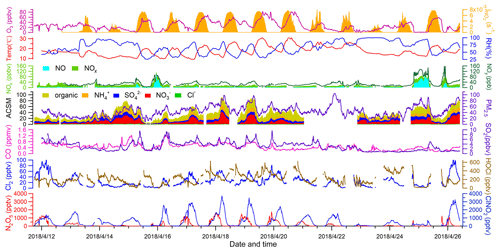Figure 2Time series of ClNO2, Cl2, and related measurements during field observations from 11 to 26 April 2018. Data gaps were caused by technical problems or calibrations.

## 3.2 High-ClNO2 cases

Figure 3 shows the observation results from 17 and 18 April to further illustrate the ClNO2 formation process. This case had the highest ClNO2 observed during the campaign and shows an example of high ClNO2 mixing ratios after midnight. As shown in Fig. 3a, the mixing ratio of ClNO2 began to increase after sunset (18:00 LT on 17 April) and decreased after midnight. The period between 22:00 and 24:00 LT on 17 April was noted as plume 1. After midnight, the ClNO2 mixing ratios exhibited a more rapid increase from 03:00 to 05:00 LT on 18 April (plume 3), and the particulate nitrate concentration also synchronously and significantly increased. Plumes 1 and 3 were identified as being different, resulting from an air mass shift between 00:00 and 03:00 LT on 18 April (plume 2), as indicated by abrupt changes in the RH, temperature, and O3. We compared the backward trajectories from plume 1 to plume 3 and found no significant difference (figures not shown here). Thus, the change in the air mass from plume 1 to plume 3 was likely a local phenomenon.

The P(NO3) and NO3 loss pathways during plumes 1 and 3 were calculated and compared in Fig. 3b–d using the methods described in Sect. 2.4. The P(NO3) was slightly lower during plume 3 than during plume 1, and a larger proportion of NO3 was lost via the N2O5 hydrolysis pathway in plume 3. Thus, the air mass shift, in addition to the higher rate of N2O5 hydrolysis, was responsible for the elevated ClNO2 levels observed after midnight.

Compared with the high levels of ClNO2 (up to 3.5 ppbv) on the night of 17 April, the concentration of Cl was low and relatively constant (∼0.1 ppbv) during that period. The low chloride but high ClNO2 levels were also observed in previous studies, and HCl partition was proposed to replenish particulate chloride to sustain the ClNO2 production (Osthoff et al., 2008; Thornton et al., 2010).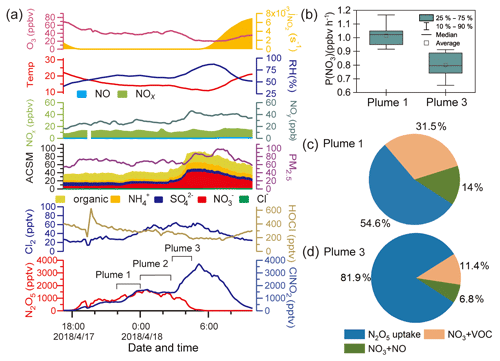Figure 3Detailed analysis of a high-ClNO2 episode observed on 17–18 April. (a) Time series of ClNO2 and related species. (b)(d) Comparisons of P(NO3) and NO3 loss pathways in plumes 1 and 3.

## 3.3ClNO2 production yield from N2O5 uptake

φ (ClNO2) was estimated to investigate its influencing factors and the performance of parameterization in selected cases. The methods described in Sect. 2.5 were used to estimate the φ (ClNO2) and γ (N2O5) using the observation data. As these methods assume a stable air mass and the dominance of N2O5 uptake in nitrate formation, we applied the following criteria when selecting cases for this analysis. First, the NO mixing ratios must be less than 0.1 ppbv. When significant levels of NO were present, the N2O5 chemistry was suppressed. Second, primary pollutants such as CO, SO2, and meteorological factors (wind, temperature, and RH) were required to exhibit relatively constant levels or stable trends within the cases. Third, the ClNO2 and nitrate levels had to be correlated (R2>0.6) and show increasing trends. Fifteen cases that lasted 30 min to 3 h were selected, and 10 min averaged data were used for calculation. Figure S7 shows an example of this calculation, which corresponds to plume 1 on 17 April (Fig. 3). We then evaluated the loss pathways of NO3 in the 15 cases. The results show that the NO3+ VOCs reactions contributed less than one-third of the total NO3+N2O5 loss (e.g., Fig. 3c, d). Nocturnal total nitrate production was thus dominated by N2O5 uptake, and only a small proportion of nitrate was produced by NO3+ VOCs reactions.

The derived γ (N2O5) values ranged from 0.004 to 0.014 (mean: 0.008±0.004). The highest γ (N2O5) values (0.0135 and 0.0139) were derived between 03:00 and 05:00 LT on 18 April (i.e., plume 3 in Fig. 3), which was consistent with the rapid increase in ClNO2 mixing ratios during that period. The variations in the γ (N2O5) value depended mainly on [H2O] (R2=0.49) (see Fig. S8) but showed little correlation with other influencing factors, such as [Cl], [${\mathrm{NO}}_{\mathrm{3}}^{-}$], and VaSa (figures not shown here). The dominant influence of [H2O] on the γ (N2O5) value was also reported in previous studies (e.g., Tham et al., 2018).

The φ (ClNO2) value ranged from 0.28 to 0.89 (mean: 0.56±0.15). The φ (ClNO2) value exhibited an obvious nonlinear relationship with the [Cl] / [H2O] ratio (R2=0.52) (Fig. 4a), which is consistent with previous laboratory results (Bertram and Thornton, 2009). However, current parameterization of φ (ClNO2) based on [Cl] / [H2O] (φ (ClNO2)BT) tended to overestimate the observed φ (ClNO2) value (Fig. 4b).

Here we give two explanations for the inconsistency between the φ (ClNO2)BT and the field-derived φ (ClNO2). First, the reactivity of chloride with ${\mathrm{NO}}_{\mathrm{2}}^{+}$ (i.e., k4k3 in Eq. 8) was reduced in ambient environments due to complicated issues of the mixing state, phase state, and activity coefficient. As φ (ClNO2) is positively dependent upon [Cl], a reduction in chloride reactivity could decrease the φ (ClNO2) value in ambient particles. This explanation is supported by previous studies of γ (N2O5) (Morgan et al., 2015; McDuffie et al., 2018a), which showed that, when the enhancement effect of chloride on γ (N2O5) was neglected, the parameterized γ (N2O5) better matched the observed γ (N2O5). The second explanation deals with other unknown factors that reduce the φ (ClNO2) value. The parameterization φ (ClNO2)BT only considered the [Cl] / [H2O] ratio, not other aqueous species that could suppress φ (ClNO2), leading to the overestimation of φ (ClNO2)BT values.

Regarding the second explanation, we examined the possibility of sulfate and aerosol organics competing with [Cl] for the ${\mathrm{NO}}_{\mathrm{2}}^{+}$ intermediate (see Sect. 2.4 and Eq. 10). The statistical results show that aerosol organics could reduce φ (ClNO2) values (R2=0.41; Fig. S9b), but sulfate did not show such an influence (R2=0.08; Fig. S9a). The latter result contrasts with the finding of a recent laboratory study which indicated that both sulfate and some organics (e.g., carboxylate) suppress ClNO2 formation (Staudt et al., 2019).

By incorporating the suppression effect of aerosol organics, we performed regressions of φ (ClNO2) and obtained an improved parameterization of φ (ClNO2) (noted as φ (ClNO2)BT+Org). The parameterized φ (ClNO2)BT+Org better matches the observed φ (ClNO2) at low to median yields (0–0.75), and the R2 and slope values in the linear regression are closer to 1 (Fig. 4b). However, the parameterized φ (ClNO2)BT+Org is smaller than the observed φ (ClNO2) at high yields (0.75–0.9), which may be attributable to other unconstrained factors in the parameterization, for example mixing state and phase state issues. In Eq. (11), the factor 483 (k4k3 in Eq. 9) was adopted from Bertram and Thornton (2009), and the factor 235 (k4k5 in Eq. 9) was derived here by iterative algorithms to achieve the least-square errors between the observed and parameterized φ (ClNO2) values. Here we assumed that the observed aerosol organics were all water-soluble and reactive toward NO${}_{\mathrm{2}}^{+}$, as previous studies did (McDuffie et al., 2018a, b). The unknown water-soluble proportion of aerosol organics is factored into k5. Given that ${k}_{\mathrm{4}}/{k}_{\mathrm{3}}=\mathrm{483}$ and ${k}_{\mathrm{4}}/{k}_{\mathrm{5}}=\mathrm{235}$, k5k3 was calculated as 2.06, which suggests that the reaction rate constant of aerosol organics with ${\mathrm{NO}}_{\mathrm{2}}^{+}$ was twice that of the H2O+${\mathrm{NO}}_{\mathrm{2}}^{+}$ reaction. A recent laboratory study (Staudt et al., 2019) derived ${k}_{\mathrm{5}}/{k}_{\mathrm{3}}=\mathrm{3.7}$ for acetate, which happens to be similar to the value derived for ambient aerosol at our site.

$\begin{array}{}\text{(11)}& \mathit{\phi }\left({\mathrm{ClNO}}_{\mathrm{2}}{\right)}_{\mathrm{BT}+\mathrm{Org}}={\left(\mathrm{1}+\frac{\left[{\mathrm{H}}_{\mathrm{2}}\mathrm{O}\right]}{\mathrm{483}\left[{\mathrm{Cl}}^{-}\right]}+\frac{\left[\mathrm{Org}\right]}{\mathrm{235}\left[{\mathrm{Cl}}^{-}\right]}\right)}^{-\mathrm{1}}\end{array}$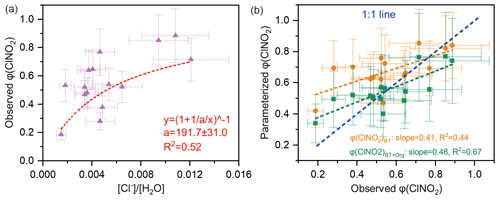Figure 4Influencing factors and parameterizations of φ (ClNO2). (a) Dependence of φ (ClNO2) on the [Cl] / [H2O] ratio. Dashed red line shows nonlinear fitting of φ (ClNO2); “a” represents the k4k3 ratio in Eq. (8). (b) Comparison of parameterized φ (ClNO2) and observed φ (ClNO2), where φ (ClNO2)BT denotes the parameterization proposed by Bertram and Thornton (2009), and φ (ClNO2)BT+Org represents the revised parameterization used in this study (see Eq. 11).

## 3.4 Nocturnal Cl2 formation

### 3.4.1Cl2 as a co-product of ClNO2 from N2O5 uptake

To elucidate the formation pathways of the elevated levels of Cl2 observed during the night, we investigated the correlations of Cl2 with the ClNO2, HOCl, and SO2 and the diurnal variations of these species (Fig. 5a–d). Our result suggests that Cl2 was related to ClNO2, but the HOCl pathway (Reaction R5) and coal burning were of minor importance at our site. ClONO2 was not measured during our study. Recent field measurements at a rural site in northern China reported low ClONO2 levels at night (maximum ∼15 pptv) (Breton et al., 2018). We believe that the ClONO2 levels at our site were also low, and production pathway (Reaction R6) was insignificant given low γ (ClONO2) ($\sim {\mathrm{10}}^{-\mathrm{3}}$) (Haskins et al., 2019). At our site, the Cl2∕ClNO2 ratios varied on different nights, which implies that differences exist in the production efficiencies of Cl2 relative to those of ClNO2.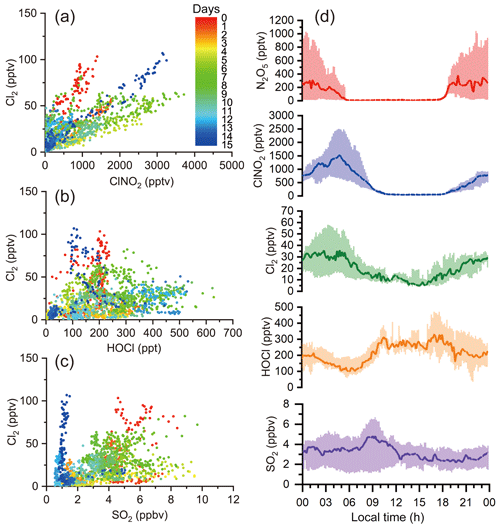Figure 5Correlations among Cl2, ClNO2, HOCl, and SO2 and their diurnal profiles; (a), (b), and (c) show the correlations of Cl2 with ClNO2, HOCl, and SO2 respectively, during the whole campaign. Dots represent 10 min averaged values colored according to campaign days. (d) exhibits the diurnal variation of Cl2, ClNO2, HOCl, and SO2.

The current mainstream interpretation of the observed correlation of ClNO2 and Cl2 is that Cl2 is produced from ClNO2 uptake (Ammann et al., 2013; Qiu et al., 2019; Wang et al., 2019; Haskins et al., 2019). We provide evidence that this interpretation does not apply to measurements from our site. We assessed the ClNO2 uptake hypothesis by examining the magnitude of γ (ClNO2) needed to explain the nocturnal increase in Cl2 mixing ratios and the dependence of γ(ClNO2) on its known influencing factors. Assuming a unity yield of Cl2 from ClNO2 uptake, the increasing rate of Cl2 mixing ratios was calculated with Eq. (12). Equation (13), which was derived by rearrangement of Eq. (12), was adopted to estimate γ (ClNO2) via the observed Cl2 and ClNO2 levels.

$\begin{array}{}\text{(12)}& d\left[{\mathrm{Cl}}_{\mathrm{2}}\right]/\mathrm{d}t=\frac{\mathrm{1}}{\mathrm{4}}c\left({\mathrm{ClNO}}_{\mathrm{2}}\right){S}_{\mathrm{a}}\mathit{\gamma }\left({\mathrm{ClNO}}_{\mathrm{2}}\right)\left[{\mathrm{ClNO}}_{\mathrm{2}}\right],\text{(13)}& \mathit{\gamma }\left({\mathrm{ClNO}}_{\mathrm{2}}{\right)}_{\mathrm{obs}}=\frac{\mathrm{4}d\left[{\mathrm{Cl}}_{\mathrm{2}}\right]/\mathrm{d}t}{c\left({\mathrm{ClNO}}_{\mathrm{2}}\right){S}_{\mathrm{a}}\left[{\mathrm{ClNO}}_{\mathrm{2}}\right]},\end{array}$

where c(ClNO2) is the mean molecular velocity of ClNO2 (m s−1), and [ClNO2] represents the averaged ambient concentration of ClNO2 in the cases of interest.

γ (ClNO2)obs was estimated in the selected cases following criteria 1 and 2 in Sect. 3.3, and a steady increase in Cl2 mixing ratios was required. The resulting values of γ (ClNO2)obs were compiled according to the local time and are presented in box charts (Fig. 6a). Figure 6a also shows the potential factors influencing γ (ClNO2): [Cl], [H+], and particle diameter (Dp). Here, Dp was an influencing factor of γ (ClNO2) because ClNO2 uptake was regarded as a volume-limited mechanism (Ammann et al., 2013; Haskins et al., 2019). [H+] and [Cl] were considered because the previous laboratory study proposed that H+ and Cl were reactants in Cl2 production (Roberts et al., 2008). Positive correlations of γ (ClNO2) with [Cl] and Dp were also found in a field study (Haskins et al., 2019). Each box represents the γ (ClNO2), [Cl], [H+], or Dp of 10 min resolutions derived on individual days. For example, the box for 18:00–19:00 contains the γ (ClNO2) estimated at 18:00–19:00 LT on 11, 12, and 14 April (Fig. 6b–d, orange lines). Figure 6b–d display the observed Cl2 levels (blue lines) and the projected trends of Cl2 levels from Eq. (12), where the grey lines adopted the highest γ (ClNO2) value, $\mathrm{6.69}×{\mathrm{10}}^{-\mathrm{5}}$, observed in the field study of Haskins et al. (2019). During early evening hours (i.e., 18:00–19:00), the γ (ClNO2) value derived in our study was 1–2 orders of magnitude higher than those in that study. This result implies that either ClNO2 uptake was much faster at our site or other pathways were involved in Cl2 production. We provide evidence below that the latter is likely the case.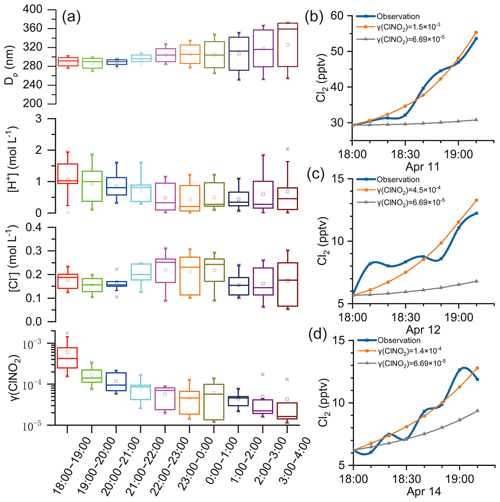Figure 6γ (ClNO2) estimated using field observation data. (a) γ (ClNO2)obs, [Cl], [H+], and Dp estimated at various nighttime periods. (b)(d) Trends of increasing trends of Cl2 mixing ratios during the early evening hours on 11, 12, and 14 April, respectively. Orange and grey lines represent the projected trend of Cl2 mixing ratios using Eq. (12) with constant γ (ClNO2) values and observed ClNO2 levels.

If the ClNO2 uptake were the main production channel for Cl2, we would expect to see positive correlations between γ (ClNO2) and factors such as [Cl], [H+], and Dp, according to previous laboratory and field studies (Roberts et al., 2008; Haskins et al., 2019). At our site, as the increasing rate of Cl2 concentrations (d[Cl2]∕dt) did not change significantly during the night (Fig. 5d), the γ (ClNO2) value was constrained by a sharp decreasing trend to compensate for the increasing ClNO2 levels after dusk (see Eq. 12). The highest γ (ClNO2)obs value determined during the early evening hours (18:00–19:00) was similar to the laboratory-derived γ (ClNO2)obs value on acidic salt films ($\mathrm{6}×{\mathrm{10}}^{-\mathrm{3}}$) (Roberts et al., 2008). However, the lowest γ (ClNO2)obs value estimated during later nighttime hours (22:00–04:00) was 2 orders of magnitude lower (10−5). The large variations in the γ (ClNO2) value contrasted with the relatively stable levels of [Cl], [H+], and Dp at various times of night, which is in opposition to the current understanding of the relationship between the γ (ClNO2) and these factors. In our study, the Dp was derived from the ratio of wet Va to Sa by assuming volume-limited uptake (Ammann et al., 2013). We also calculated Dp assuming surface-limited uptake (diameter of the average surface area), and no correlation with γ (ClNO2)obs was indicated. Moreover, the γ (ClNO2)obs showed no obvious relationship with other factors such as T, RH, aerosol liquid water content (ALW), ${\mathrm{NO}}_{\mathrm{3}}^{-}$, ${\mathrm{SO}}_{\mathrm{4}}^{\mathrm{2}-}$, ${\mathrm{NH}}_{\mathrm{4}}^{+}$, and aerosol organics (figure not shown). To sum up, the ClNO2 uptake pathway alone cannot explain the nocturnal increase in Cl2 mixing ratios that we observed at our study site.

We propose another hypothesis to explain the ClNO2Cl2 correlation and suggest that Cl2 is a co-product with ClNO2 produced from N2O5 uptake, in which ClNO2 is not necessarily an intermediate of Cl2 production. The mechanism is depicted in Fig. 7 and goes as follows. It is known that N2O5 hydrolysis on aerosol is responsible for the production of ${\mathrm{NO}}_{\mathrm{2}}^{+}$. According to the hybrid orbital theory, the ${\mathrm{NO}}_{\mathrm{2}}^{+}$ ion has two non-bonded π molecular orbitals due to participation of the d orbital of the central nitrogen atom (Baird and Tayler, 1981). ClNO2 is formed via the nucleophilic addition of Cl to one of the π molecular orbitals of ${\mathrm{NO}}_{\mathrm{2}}^{+}$ (Fig. 7a) (Taylor, 1990; Behnke et al., 1997). In the same way, we propose a side reaction: the second Cl can attach to the other π molecular orbital of ${\mathrm{NO}}_{\mathrm{2}}^{+}$ and form a short-lived HNO2Cl2 intermediate in the presence of H+. It is proposed that the unstable HNO2Cl2 decomposes to produce Cl2 (and HONO) (Fig. 7b). This mechanism can explain concurrent productions of Cl2 and ClNO2 from N2O5 hydrolysis but needs confirmation by additional laboratory and theoretical studies.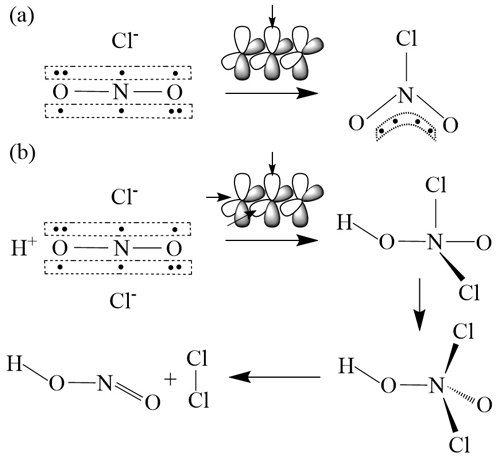Figure 7Proposed formation mechanisms of ClNO2 and Cl2 from N2O5 uptake. (a) Production of ClNO2 from ${\mathrm{NO}}_{\mathrm{2}}^{+}$ and Cl. (b) Production of Cl2 from ${\mathrm{NO}}_{\mathrm{2}}^{+}$, Cl, and H+.

### 3.4.2 Parameterizing Cl2 formation from N2O5 uptake

We propose a new framework to estimate nighttime Cl2 production by treating Cl2, ClNO2, and most nitrate all ultimately originating from N2O5 uptake. We assign a production yield to Cl2 from the N2O5 uptake (φ (Cl2)) analogous to the ClNO2 yield and calculate this metric using Eq. (14):

$\begin{array}{}\text{(14)}& \mathit{\phi }\left({\mathrm{Cl}}_{\mathrm{2}}\right)=\frac{d\left[{\mathrm{Cl}}_{\mathrm{2}}\right]/\mathrm{d}t}{k\left({\mathrm{N}}_{\mathrm{2}}{\mathrm{O}}_{\mathrm{5}}\right)\left[{\mathrm{N}}_{\mathrm{2}}{\mathrm{O}}_{\mathrm{5}}\right]}.\end{array}$

The above formulation does not rule out the production of Cl2 from the ClNO2 uptake, because such production, if any, is also a result of N2O5 uptake and has thus been incorporated in Eq. (14). We calculated φ (Cl2) in the same cases in which γ (N2O5) and φ (ClNO2) were derived, because the availability of γ (N2O5) was a prerequisite of deriving φ (Cl2). The estimated φ (Cl2) value was 0.01–0.04 (Table S2). The dependences of φ (Cl2) on its potential influencing factors (i.e., [Cl], [H+], and Dp) were examined. The results show that φ (Cl2) had positive correlations with both [Cl] (R2=0.74) and [H+] (R2=0.75) and that the data had a high-φ (Cl2) region and a low-φ (Cl2) region (Fig. 8a, b). The low φ (Cl2) values were found in continental air masses with relatively lower chloride concentrations, more alkaline ammonium, less acidic sulfate and nitrate, and thus lower acidity (Fig. 8d). In contrast, the high φ (Cl2) values were associated with marine air masses with higher loadings of aerosol chloride, less ammonium, and more acidic compounds, and thus higher acidity (Fig. 8c). The higher acidity in the marine air masses may be explained by their passage over the industrialized cities in the YRD where large amount of SO2 and NOx are emitted. The average concentrations of SO2 (3.9±0.1 ppbv) and NOx (13.1±3.1 ppbv) in the marine air masses were higher than those (NOx: 11.5±0.6 ppbv; SO2: 3.3±0.3 ppbv) in the inland air masses. The dependences of the defined φ (Cl2) on [Cl] and [H+] indicate that nocturnal Cl2 production requires the presence of highly acidic chloride-rich particles and sufficient levels of N2O5.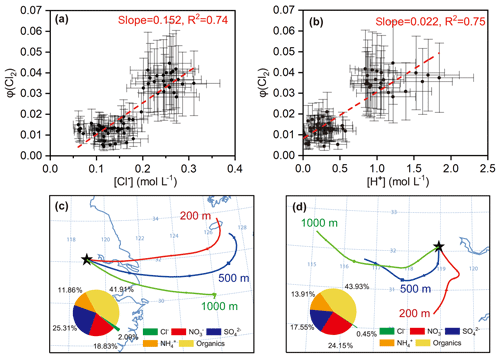Figure 8Estimated φ (Cl2) from N2O5 uptake and the factors influencing φ (Cl2) (a) and (b) dependencies of φ (Cl2) on [Cl] and [H+] in selected cases. (c) and (d) are examples of high φ (Cl2) values in marine air masses (e.g., 13 April) and low φ (Cl2) values in inland air masses (e.g., 18 April) represented by 24 h backward trajectories (see Fig. S2 for trajectories during the whole observations). Inserted pie charts show average aerosol chemical compositions during 21:40 LT on 12 April to 00:40 LT on 13 April and from 22:20 to 23:40 LT on 17 April, respectively.

A parameterization scheme is derived based on the dependences of φ (Cl2) on [Cl] and [H+] to predict the Cl2 formation involving N2O5 heterogeneous chemistry. Mechanistically, it is assumed that the nocturnal Cl2 is produced from reactions involving ${\mathrm{NO}}_{\mathrm{2}}^{+}$ that can be produced either from uptake of N2O5 or ClNO2. The production rates of nitrate, ClNO2, and Cl2 from the loss of ${\mathrm{NO}}_{\mathrm{2}}^{+}$ are expressed in Eq. (15) through Eq. (17). The loss rate of aerosol organics induced by ${\mathrm{NO}}_{\mathrm{2}}^{+}$ is expressed in Eq. (18) (noted as d[Org]/dt here).

$\begin{array}{}\text{(15)}& d\left[{\mathrm{NO}}_{\mathrm{3}}^{-}\right]/\mathrm{d}t={k}_{\mathrm{3}}\left[{\mathrm{NO}}_{\mathrm{2}}^{+}\right]\left[{\mathrm{H}}_{\mathrm{2}}\mathrm{O}\right]\text{(16)}& d\left[{\mathrm{ClNO}}_{\mathrm{2}}\right]/\mathrm{d}t={k}_{\mathrm{4}}\left[{\mathrm{NO}}_{\mathrm{2}}^{+}\right]\left[{\mathrm{Cl}}^{-}\right]\text{(17)}& d\left[{\mathrm{Cl}}_{\mathrm{2}}\right]/\mathrm{d}t={k}_{\mathrm{6}}\left[{\mathrm{NO}}_{\mathrm{2}}^{+}\right]\left[{\mathrm{Cl}}^{-}\right]\left[{\mathrm{H}}^{+}\right]\text{(18)}& d\left[\mathrm{Org}\right]/\mathrm{d}t={k}_{\mathrm{5}}\left[{\mathrm{NO}}_{\mathrm{2}}^{+}\right]\left[\mathrm{Org}\right]\end{array}$

The symbol k6 represents the rate constant of the reaction involving ${\mathrm{NO}}_{\mathrm{2}}^{+}$, Cl, and H+. φ (Cl2) is obtained as follows, by assuming a steady state of the ${\mathrm{NO}}_{\mathrm{2}}^{+}$ intermediate (Bertram and Thornton, 2009) (Eq. 19).

$\begin{array}{}\text{(19)}& \begin{array}{rl}\mathit{\phi }& \left({\mathrm{Cl}}_{\mathrm{2}}\right)=\frac{\frac{d\left[{\mathrm{Cl}}_{\mathrm{2}}\right]}{\mathrm{d}t}}{\frac{d\left[{\mathrm{Cl}}_{\mathrm{2}}\right]}{\mathrm{d}t}+\frac{d\left[{\mathrm{ClNO}}_{\mathrm{2}}\right]}{\mathrm{d}t}+\frac{d\left[{{\mathrm{NO}}_{\mathrm{3}}}^{-}\right]}{\mathrm{d}t}+\frac{d\left[\mathrm{Org}\right]}{\mathrm{d}t}}\\ & =\frac{{k}_{\mathrm{6}}\left[{\mathrm{Cl}}^{-}\right]\left[{\mathrm{H}}^{+}\right]}{{k}_{\mathrm{6}}\left[{\mathrm{Cl}}^{-}\right]\left[{\mathrm{H}}^{+}\right]+{k}_{\mathrm{4}}\left[{\mathrm{Cl}}^{-}\right]+{k}_{\mathrm{3}}\left[{\mathrm{H}}_{\mathrm{2}}\mathrm{O}\right]+{k}_{\mathrm{5}}\left[\mathrm{Org}\right]}\end{array}\end{array}$

To remain consistent with the φ (ClNO2) parameterization, the values 483 and 2.06 were adopted for k4k3 and k5k3, respectively, while k6k3 was estimated from the fitting of φ (Cl2) using Eq. (19) to achieve the least-squares errors between the observed and parameterized φ (Cl2) values. The parameterization of φ (Cl2) was then expressed as follows (Eq. 20):

$\begin{array}{}\text{(20)}& \begin{array}{rl}& \mathit{\phi }\left({\mathrm{Cl}}_{\mathrm{2}}\right)=\\ & \frac{\mathrm{19.38}\left[{\mathrm{H}}^{+}\right]\left[{\mathrm{Cl}}^{-}\right]}{\mathrm{19.38}\left[{\mathrm{H}}^{+}\right]\left[{\mathrm{Cl}}^{-}\right]+\mathrm{483}\left[{\mathrm{Cl}}^{-}\right]+\left[{\mathrm{H}}_{\mathrm{2}}\mathrm{O}\right]+\mathrm{2.06}\left[\mathrm{Org}\right]},\end{array}\end{array}$

where the unit of [H+], [Cl], and [Org] is mole per liter (mol L−1).

The previous ClNO2 uptake method assumed a unity Cl2 yield from ClNO2 uptake, but no such assumption is required in the new method for an explicit definition (Eq. 14) and parameterization (Eq. 20) of the φ (Cl2). In addition, a quantitative relationship between φ (Cl2) and aerosol acidity is established, which was not given in the previous parameterization. We recommend that air quality models test this parameterization for reproduction of nighttime Cl2 observations.

4 Summary and conclusions

This study reports the presence of significant levels of ClNO2 and Cl2 at a suburban site in eastern China. A rapid increase in the ClNO2 mixing ratios was found to occur after midnight due to larger rates of N2O5 heterogeneous loss than in early nighttime hours, and a high φ (ClNO2) value was also responsible for the elevated ClNO2 mixing ratios. Improved parameterization of φ (ClNO2) at low to moderate range was achieved by involving the suppression effect of aerosol organics. We propose that the observed nighttime Cl2 was co-produced with ClNO2 from the heterogeneous N2O5 uptake on acidic aerosols that bore chloride and suggest a mechanism for simultaneous production of ClNO2 and Cl2 from N2O5 hydrolysis. We have proposed a parameterization for φ (Cl2) from N2O5 uptake. The combination of φ (Cl2), φ (ClNO2), and γ (N2O5) can be used in air quality models to predict the nighttime formation of Cl2 and ClNO2 from N2O5 uptake and their effect on the next day's atmospheric photochemistry.

Data availability
Data availability.

To request the CIMS, jNO2, and NOy data described in this study, please contact the corresponding author (cetwang@polyu.edu.hk). Other datasets are available by contacting Wei Nie (niewei@nju.edu.cn).

Supplement
Supplement.

Author contributions
Author contributions.

TW designed the research. WN and AD managed the sampling sites. MX, XP, and WW performed the CIMS measurements. CY, ZX, PS, YL, YL, and ZX provided other data. MX and TW wrote the manuscript with comments from all co-authors.

Competing interests
Competing interests.

The authors declare that they have no conflict of interest.

Acknowledgements
Acknowledgements.

The authors acknowledge helpful opinions and discussions from Yee Jun Tham.

Financial support
Financial support.

This research has been supported by the National Natural Science Foundation of China (grant nos. 91544213 and D0512/41675145) and the Hong Kong Research Grants Council (grant no. T24-504/17-N).

Review statement
Review statement.

This paper was edited by Steven Brown and reviewed by two anonymous referees.

References

Ammann, M., Cox, R. A., Crowley, J. N., Jenkin, M. E., Mellouki, A., Rossi, M. J., Troe, J., and Wallington, T. J.: Evaluated kinetic and photochemical data for atmospheric chemistry: Volume VI – heterogeneous reactions with liquid substrates, Atmos. Chem. Phys., 13, 8045–8228, https://doi.org/10.5194/acp-13-8045-2013, 2013.

Baird, N. C. and Taylor, K. F.: The stabilizing effect of d orbitals on the central nitrogen atom in nitrogen-oxygen molecules and ions, Chem. Phys. Lett., 80, 83–86, https://doi.org/10.1016/0009-2614(81)80062-0, 1981.

Behnke, W., George, C., Scheer, V., and Zetzsch, C.: Production and decay of ClNO2 from the reaction of gaseous N2O5 with NaCl solution: Bulk and aerosol experiments, J. Geophys. Res.-Atmos., 102, 3795–3804, 1997.

Bertram, T. H. and Thornton, J. A.: Toward a general parameterization of N2O5 reactivity on aqueous particles: the competing effects of particle liquid water, nitrate and chloride, Atmos. Chem. Phys., 9, 8351–8363, https://doi.org/10.5194/acp-9-8351-2009, 2009.

Custard, K. D., Pratt, K. A., Wang, S., and Shepson, P. B.: Constraints on Arctic Atmospheric Chlorine Production through Measurements and Simulations of Cl2 and ClO, Environ. Sci. Technol., 50, 12394–12400, https://doi.org/10.1021/acs.est.6b03909, 2016.

Deiber, G., George, Ch., Le Calvé, S., Schweitzer, F., and Mirabel, Ph.: Uptake study of ClONO2 and BrONO2 by Halide containing droplets, Atmos. Chem. Phys., 4, 1291–1299, https://doi.org/10.5194/acp-4-1291-2004, 2004.

Ding, A. J., Fu, C. B., Yang, X. Q., Sun, J. N., Zheng, L. F., Xie, Y. N., Herrmann, E., Nie, W., Petäjä, T., Kerminen, V.-M., and Kulmala, M.: Ozone and fine particle in the western Yangtze River Delta: an overview of 1 yr data at the SORPES station, Atmos. Chem. Phys., 13, 5813–5830, https://doi.org/10.5194/acp-13-5813-2013, 2013.

Ding, A., Huang, X., Nie, W., Chi, X., Xu, Z., Zheng, L., Xu, Z., Xie, Y., Qi, X., Shen, Y., Sun, P., Wang, J., Wang, L., Sun, J., Yang, X.-Q., Qin, W., Zhang, X., Cheng, W., Liu, W., Pan, L., and Fu, C.: Significant reduction of PM2.5 in eastern China due to regional-scale emission control: evidence from SORPES in 2011–2018, Atmos. Chem. Phys., 19, 11791–11801, https://doi.org/10.5194/acp-19-11791-2019, 2019.

Finlayson-Pitts, B., Ezell, M., and Pitts, J.: Formation of chemically active chlorine compounds by reactions of atmospheric NaCl particles with gaseous N2O5 and ClONO2, Nature, 337, 241–244, 1989.

Haskins, J. D., Lee, B. H., Lopez-Hilifiker, F. D., Peng, Q., Jaeglé, L., Reeves, J. M., Schroder, J. C., Campuzano-Jost, P., Fibiger, D., and McDuffie, E. E.: Observational constraints on the formation of Cl2 from the reactive uptake of ClNO2 on aerosols in the polluted marine boundary layer, J. Geophys. Res.-Atmos., 124, 8851–8869, 2019.

Kercher, J. P., Riedel, T. P., and Thornton, J. A.: Chlorine activation by N2O5: simultaneous, in situ detection of ClNO2 and N2O5 by chemical ionization mass spectrometry, Atmos. Meas. Tech., 2, 193–204, https://doi.org/10.5194/amt-2-193-2009, 2009.

Le Breton, M., Hallquist, Å. M., Pathak, R. K., Simpson, D., Wang, Y., Johansson, J., Zheng, J., Yang, Y., Shang, D., Wang, H., Liu, Q., Chan, C., Wang, T., Bannan, T. J., Priestley, M., Percival, C. J., Shallcross, D. E., Lu, K., Guo, S., Hu, M., and Hallquist, M.: Chlorine oxidation of VOCs at a semi-rural site in Beijing: significant chlorine liberation from ClNO2 and subsequent gas- and particle-phase Cl–VOC production, Atmos. Chem. Phys., 18, 13013–13030, https://doi.org/10.5194/acp-18-13013-2018, 2018.

Lewis, E. R.: An examination of Köhler theory resulting in an accurate expression for the equilibrium radius ratio of a hygroscopic aerosol particle valid up to and including relative humidity 100 %, J. Geophys. Res.-Atmos., 113, D3, https://doi.org/10.1029/2007JD008590, 2008.

Liao, J., Huey, L. G., Liu, Z., Tanner, D. J., Cantrell, C. A., Orlando, J. J., Flocke, F. M., Shepson, P. B., Weinheimer, A. J., and Hall, S. R.: High levels of molecular chlorine in the Arctic atmosphere, Nat. Geosci., 7, 91–94, 2014.

Liu, X., Qu, H., Huey, L. G., Wang, Y., Sjostedt, S., Zeng, L., Lu, K., Wu, Y., Hu, M., and Shao, M.: High levels of daytime molecular chlorine and nitryl chloride at a rural site on the North China Plain, Environ. Sci. Technol., 51, 9588–9595, 2017.

Li, Y., Nie, W., Liu, Y., Huang, D., Xu, Z., Peng, X., George, C., Yan, C., Tham, Y. J., Yu, C., Xia, M., Fu, X., Wang, X., Xue, L., Wang, Z., Xu, Z., Chi, X., Wang, T., and Ding, A.: Photoinduced Production of Chlorine Molecules from Titanium Dioxide Surfaces Containing Chloride, Environ. Sci. Technol. Lett., 7, 70–75, https://doi.org/10.1021/acs.estlett.9b00704, 2020.

McDuffie, E. E., Fibiger, D. L., Dubé, W. P., Lopez-Hilfiker, F., Lee, B. H., Thornton, J. A., Shah, V., Jaeglé, L., Guo, H., and Weber, R. J.: Heterogeneous N2O5 uptake during winter: Aircraft measurements during the 2015 WINTER campaign and critical evaluation of current parameterizations, J. Geophys. Res.-Atmos., 123, 4345–4372, 2018a.

McDuffie, E. E., Fibiger, D. L., Dubé, W. P., Lopez Hilfiker, F., Lee, B. H., Jaeglé, L., Guo, H., Weber, R. J., Reeves, J. M., and Weinheimer, A. J.: ClNO2 yields from aircraft measurements during the 2015 WINTER campaign and critical evaluation of the current parameterization, J. Geophys. Res.-Atmos., 123, 12994–913015, 2018b.

McNamara, S. M., Raso, A. R., Wang, S., Thanekar, S., Boone, E. J., Kolesar, K. R., Peterson, P. K., Simpson, W. R., Fuentes, J. D., and Shepson, P. B.: Springtime Nitrogen Oxide-Influenced Chlorine Chemistry in the Coastal Arctic, Environ. Sci. Technol., 53, 8057–8067, 2019.

Mielke, L. H., Furgeson, A., and Osthoff, H. D.: Observation of ClNO2 in a mid-continental urban environment, Environ. Sci. Technol., 45, 8889–8896, https://doi.org/10.1021/es201955u, 2011.

Molina, M. J. and Rowland, F. S.: Stratospheric sink for chlorofluoromethanes: chlorine atom-catalysed destruction of ozone, Nature, 249, 810–812, 1974.

Morgan, W. T., Ouyang, B., Allan, J. D., Aruffo, E., Di Carlo, P., Kennedy, O. J., Lowe, D., Flynn, M. J., Rosenberg, P. D., Williams, P. I., Jones, R., McFiggans, G. B., and Coe, H.: Influence of aerosol chemical composition on N2O5 uptake: airborne regional measurements in northwestern Europe, Atmos. Chem. Phys., 15, 973–990, https://doi.org/10.5194/acp-15-973-2015, 2015.

Osthoff, H. D., Roberts, J. M., Ravishankara, A. R., Williams, E. J., Lerner, B. M., Sommariva, R., Bates, T. S., Coffman, D., Quinn, P. K., Dibb, J. E., Stark, H., Burkholder, J. B., Talukdar, R. K., Meagher, J., Fehsenfeld, F. C., and Brown, S. S.: High levels of nitryl chloride in the polluted subtropical marine boundary layer, Nat. Geosci., 1, 324–328, https://doi.org/10.1038/ngeo177, 2008.

Phillips, G. J., Thieser, J., Tang, M., Sobanski, N., Schuster, G., Fachinger, J., Drewnick, F., Borrmann, S., Bingemer, H., Lelieveld, J., and Crowley, J. N.: Estimating N2O5 uptake coefficients using ambient measurements of NO3, N2O5, ClNO2 and particle-phase nitrate, Atmos. Chem. Phys., 16, 13231–13249, https://doi.org/10.5194/acp-16-13231-2016, 2016.

Pratte, P. and Rossi, M. J.: The heterogeneous kinetics of HOBr and HOCl on acidified sea salt and model aerosol at 40–90 % relative humidity and ambient temperature, Phys. Chem. Chem. Phys., 8, 3988–4001, 2006.

Priestley, M., le Breton, M., Bannan, T. J., Worrall, S. D., Bacak, A., Smedley, A. R. D., Reyes-Villegas, E., Mehra, A., Allan, J., Webb, A. R., Shallcross, D. E., Coe, H., and Percival, C. J.: Observations of organic and inorganic chlorinated compounds and their contribution to chlorine radical concentrations in an urban environment in northern Europe during the wintertime, Atmos. Chem. Phys., 18, 13481–13493, https://doi.org/10.5194/acp-18-13481-2018, 2018.

Qiu, X., Ying, Q., Wang, S., Duan, L., Zhao, J., Xing, J., Ding, D., Sun, Y., Liu, B., Shi, A., Yan, X., Xu, Q., and Hao, J.: Modeling the impact of heterogeneous reactions of chlorine on summertime nitrate formation in Beijing, China, Atmos. Chem. Phys., 19, 6737–6747, https://doi.org/10.5194/acp-19-6737-2019, 2019.

Riedel, T. P., Bertram, T. H., Crisp, T. A., Williams, E. J., Lerner, B. M., Vlasenko, A., Li, S. M., Gilman, J., de Gouw, J., Bon, D. M., Wagner, N. L., Brown, S. S., and Thornton, J. A.: Nitryl chloride and molecular chlorine in the coastal marine boundary layer, Environ. Sci. Technol., 46, 10463–10470, https://doi.org/10.1021/es204632r, 2012.

Riedel, T. P., Wagner, N. L., Dubé, W. P., Middlebrook, A. M., Young, C. J., Öztürk, F., Bahreini, R., VandenBoer, T. C., Wolfe, D. E., and Williams, E. J.: Chlorine activation within urban or power plant plumes: Vertically resolved ClNO2 and Cl2 measurements from a tall tower in a polluted continental setting, J. Geophys. Res.-Atmos., 118, 8702–8715, 2013.

Roberts, J. M., Osthoff, H. D., Brown, S. S., and Ravishankara, A.: N2O5 oxidizes chloride to Cl2 in acidic atmospheric aerosol, Science, 321, 1059–1059, 2008.

Roberts, J. M., Osthoff, H. D., Brown, S. S., Ravishankara, A., Coffman, D., Quinn, P., and Bates, T.: Laboratory studies of products of N2O5 uptake on Cl containing substrates, Geophys. Res. Lett., 36, 20, https://doi.org/10.1029/2009GL040448, 2009.

Seinfeld, J. H. and Pandis, S. N.: Atmospheric chemistry and physics: from air pollution to climate change, John Wiley & Sons, 2016.

Simpson, W. R., Brown, S. S., Saiz-Lopez, A., Thornton, J. A., and von Glasow, R.: Tropospheric halogen chemistry: Sources, cycling, and impacts, Chem. Rev., 115, 4035–4062, 2015.

Spicer, C., Chapman, E., Finlayson-Pitts, B., Plastridge, R., Hubbe, J., Fast, J., and Berkowitz, C.: Unexpectedly high concentrations of molecular chlorine in coastal air, Nature, 394, 353–356, 1998.

Staudt, S., Gord, J. R., Karimova, N., McDuffie, E. E., Brown, S. S., Gerber, R. B., Nathanson, G. M., and Bertram, T. H.: Sulfate and Carboxylate Suppress the Formation of ClNO2 at Atmospheric Interfaces, ACS Earth Space Chem., 3, 1987–1997, 2019.

Sun, P., Nie, W., Chi, X., Xie, Y., Huang, X., Xu, Z., Qi, X., Xu, Z., Wang, L., Wang, T., Zhang, Q., and Ding, A.: Two years of online measurement of fine particulate nitrate in the western Yangtze River Delta: influences of thermodynamics and N2O5 hydrolysis, Atmos. Chem. Phys., 18, 17177–17190, https://doi.org/10.5194/acp-18-17177-2018, 2018.

Taylor, R., Electrophilic Aromatic Substitution, John Wiley, New York, 1990.

Tham, Y. J., Wang, Z., Li, Q., Yun, H., Wang, W., Wang, X., Xue, L., Lu, K., Ma, N., Bohn, B., Li, X., Kecorius, S., Größ, J., Shao, M., Wiedensohler, A., Zhang, Y., and Wang, T.: Significant concentrations of nitryl chloride sustained in the morning: investigations of the causes and impacts on ozone production in a polluted region of northern China, Atmos. Chem. Phys., 16, 14959–14977, https://doi.org/10.5194/acp-16-14959-2016, 2016.

Tham, Y. J., Wang, Z., Li, Q., Wang, W., Wang, X., Lu, K., Ma, N., Yan, C., Kecorius, S., Wiedensohler, A., Zhang, Y., and Wang, T.: Heterogeneous N2O5 uptake coefficient and production yield of ClNO2 in polluted northern China: roles of aerosol water content and chemical composition, Atmos. Chem. Phys., 18, 13155–13171, https://doi.org/10.5194/acp-18-13155-2018, 2018.

Thornton, J. A., Braban, C. F., and Abbatt, J. P.: N2O5 hydrolysis on sub-micron organic aerosols: The effect of relative humidity, particle phase, and particle size, Phys. Chem. Chem. Phys., 5, 4593–4603, 2003.

Thornton, J. A., Kercher, J. P., Riedel, T. P., Wagner, N. L., Cozic, J., Holloway, J. S., Dube, W. P., Wolfe, G. M., Quinn, P. K., Middlebrook, A. M., Alexander, B., and Brown, S. S.: A large atomic chlorine source inferred from mid-continental reactive nitrogen chemistry, Nature, 464, 271–274, https://doi.org/10.1038/nature08905, 2010.

Wang, T., Tham, Y. J., Xue, L., Li, Q., Zha, Q., Wang, Z., Poon, S. C., Dubé, W. P., Blake, D. R., and Louie, P. K.: Observations of nitryl chloride and modeling its source and effect on ozone in the planetary boundary layer of southern China, J. Geophys. Res.-Atmos., 121, 2476–2489, 2016.

Wang, Z., Wang, W., Tham, Y. J., Li, Q., Wang, H., Wen, L., Wang, X., and Wang, T.: Fast heterogeneous N2O5 uptake and ClNO2 production in power plant and industrial plumes observed in the nocturnal residual layer over the North China Plain, Atmos. Chem. Phys., 17, 12361–12378, https://doi.org/10.5194/acp-17-12361-2017, 2017.

Wang, X., Jacob, D. J., Eastham, S. D., Sulprizio, M. P., Zhu, L., Chen, Q., Alexander, B., Sherwen, T., Evans, M. J., Lee, B. H., Haskins, J. D., Lopez-Hilfiker, F. D., Thornton, J. A., Huey, G. L., and Liao, H.: The role of chlorine in global tropospheric chemistry, Atmos. Chem. Phys., 19, 3981–4003, https://doi.org/10.5194/acp-19-3981-2019, 2019.

Wexler, A. S.: Atmospheric aerosol models for systems including the ions H+, ${\mathrm{NH}}_{\mathrm{4}}^{+}$, Na+, ${\mathrm{SO}}_{\mathrm{4}}^{\mathrm{2}-}$, ${\mathrm{NO}}_{\mathrm{3}}^{-}$, Cl, Br, and H2O, J. Geophys. Res., 107, ACH14-11–ACH14-14, https://doi.org/10.1029/2001jd000451, 2002.

Yun, H., Wang, W., Wang, T., Xia, M., Yu, C., Wang, Z., Poon, S. C. N., Yue, D., and Zhou, Y.: Nitrate formation from heterogeneous uptake of dinitrogen pentoxide during a severe winter haze in southern China, Atmos. Chem. Phys., 18, 17515–17527, https://doi.org/10.5194/acp-18-17515-2018, 2018.

Zhou, Y., Zhao, Y., Mao, P., Zhang, Q., Zhang, J., Qiu, L., and Yang, Y.: Development of a high-resolution emission inventory and its evaluation and application through air quality modeling for Jiangsu Province, China, Atmos. Chem. Phys., 17, 211–233, https://doi.org/10.5194/acp-17-211-2017, 2017.